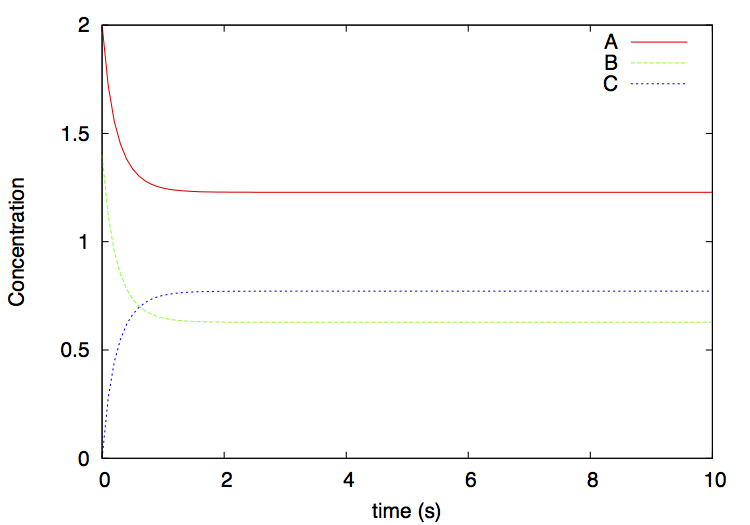[top] [up] [previous] [next]PyCellChemistry: The Dimer Example

The Dimer.py example implements a simple reversible dimerization reaction:

where C is a dimer of monomers A and B. This is an example of nonconstructive chemistry where the set of molecules S and the set of reactions R are defined explicitly. In PyCellChemistry such ACs are specified simply by the set R and the initial amount of chemicals present (S0). It is not necessary to enumerate all possible molecules in S, since molecules that don't react don't participate in the dynamics of the system, and therefore don't need to be explicitly included in the simulation. The choice of the algorithm A determines the level of resolution at which we observe the system: The same chemistry (R, S0) can be observed at a smaller scale by choosing A as a stochastic simulator, and at a coarser scale by choosing A as an ODE integrator.

Understanding the Python code

We start by defining the set of reactions R, together with their kinetic coefficients k (lines 4-7 of figure 1):

reactionstrs = [
"A + B --> C , k=1",
"C --> A + B , k=1"
]

Note that each direction of a reversible reaction must be specified separately. If a kinetic coefficient is not specified for a reaction, its default value is one. Hence, this reaction set can also be written simply as:

reactionstrs = [
"A + B --> C",
"C --> A + B"
]

This set of reactions is specified as a human-readable list of strings reactionstrs that must be parsed so that they can be more efficiently processed by the chosen reaction algorithm.

For this example we have chosen an ODE integrator implemented by the WellStirredVessel class (within ReactionVessel.py). Therefore, we now create a reaction algorithm object reactor, which is an instance of the WellStirredVessel class, and use it to parse the reaction list reactionstrs (lines 8-9 of figure 1):

self.reactor = WellStirredVessel()
self.reactor.parse(reactionstrs)

The parse method calls the parser in ReactionParser.py to parse the list reactionstrs, adds the resulting Reaction objects to the reactor, and closes the system of reactions such that it is ready to be used for ODE integration. From this point on, no further reactions may be added to the system (or at least it is not straightforward to do so).

Before we start the integration, we deposit some initial amount of substances A and B in the reactor (lines 10-11 of figure 1):

self.reactor.deposit('A', 2.0)
self.reactor.deposit('B', 1.4)

The method deposit(m, c) deposits a concentration c (in mols per unit of volume) of molecule m in the vessel.

The amount of substance C is not specified; therefore, it is considered as zero.

We now run the algorithm by invoking the integrate method at regular intervals dt, until the final simulation time finalvt is reached (lines 13-16 of figure 1):

self.reactor.trace_title()
while (self.reactor.vtime() <= finalvt):
self.reactor.trace_conc()
self.reactor.integrate(dt)

In this case, we would like to plot the concentrations of substances over time, so we invoke trace methods for that: trace_title prints a title line containing the names of the substances (in this case, 'A', 'B', and 'C') separated by tabs; trace_conc prints a line with the concentrations of each substance in the system, also separated by tabs. The output is written to stdout by default, so it will appear on the screen, but it can also be redirected to a file such that it can be plotted with a plotting tool such as gnuplot or other.

Finally, we bootstrap the system by creating an object of class Dimerization, and then run it with the method run (lines 18-19 of figure 1):

dimer = Dimerization()
dimer.run()

(Footnote: line 17 ensures that the Dimer code is only executed when invoked directly as a script, not when it is included from another script; this check is needed to avoid Python from executing this code when generating the HTML reference documentation automatically with pydoc)

Running the example

You can now run this program by invoking it from Python. From a Unix command line shell:

cd pycellchem-2.0/src
python Dimer.py > dimer.gr

This will produce a tab-separated file dimer.gr with the concentrations of A, B, and C over time. You will see that the system reaches an equilibrium in which the concentrations of the 3 substances no longer change (figure 2).

Running it from a shell script

Another way to run this example is to invoke the dimer.sh shell script found in the src/scripts folder:

cd pycellchem-2.0/src
./scripts/dimer.sh

Note that the dimer.sh script is located in the src/scripts folder, but it must be involked from the src/ folder where the examples are located.

This script invokes Python as before, and plots the output file dimer.gr using gnuplot. A file dimer.eps is produced: it depicts the concentrations over time of the various substances. This file can be visualized by simply clicking on it, or from the command line on MacOS with

open dimer.eps

and on Linux with tools such as gv or gimp.

The result is:Figure 2: output of the dimer example, as plotted by the dimer.sh script.

Modifying the example

It is now up to you to modify the example as you wish, for instance, by changing the various parameters or by adding more reactions and more chemicals to the system. You can also modify the system to run a stochastic simulation instead of an ODE integration.Last updated: July 14, 2015, by Lidia Yamamoto